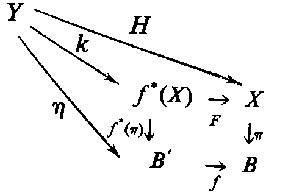# Induced fibre bundle

(diff) ← Older revision | Latest revision (diff) | Newer revision → (diff)

induced fibration

The fibration $f ^ { * } ( \pi ) : X ^ \prime \rightarrow B ^ \prime$ induced by the mapping $f : B ^ \prime \rightarrow B$ and the fibration $\pi : X \rightarrow B$, where $X ^ \prime$ is the subspace of the direct product $B ^ \prime \times X$ consisting of the pairs $( b ^ \prime , x )$ for which $f ( b ^ \prime ) = \pi ( x)$, and $f ^ { * } ( x)$ is the mapping defined by the projection $( b ^ \prime , x ) \rightarrow b ^ \prime$. The mapping $F : f ^ { * } ( X) \rightarrow X$ from the induced fibre bundle into the original fibre bundle defined by the formula $F ( b ^ \prime , x ) = x$ is a bundle morphism covering $f$. For each point $b ^ \prime \in B$, the restrictions

$$F _ {b ^ \prime } : \ ( f ^ { * } ( \pi ) ) ^ {- 1} ( b ^ \prime ) \rightarrow \pi ^ {- 1} ( f ( b ^ \prime ) )$$

are homeomorphisms. Furthermore, for any fibration $\eta : Y \rightarrow B ^ \prime$ and morphism $H : \eta \rightarrow \pi$ covering $f$ there exist precisely one $B ^ \prime$-morphism $K : \eta \rightarrow f ^ { * } ( \pi )$ such that $F K = H$, $f ^ { * } ( \pi ) K= \eta$ and such that the following diagram is commutative: $Y$ $H$ $k$ $\eta$Figure: i050720a

$$\begin{array}{ccl} f ^ { * } ( X) &\rightarrow ^ { F } & X \\ size - 3 {f ^ { * } ( \pi ) } \downarrow &{} &\downarrow size - 3 \pi \\ B ^ \prime &\rightarrow _ { f } & B \\ \end{array}$$

Fibre bundles induced from isomorphic fibrations are isomorphic, a fibre bundle induced by a constant mapping is isomorphic to the trivial fibre bundle.

For any section of a fibration $\pi$, the mapping $\sigma : B ^ \prime \rightarrow f ^ { * } ( x)$ defined by the formula $\sigma ( b ^ \prime ) = ( b ^ \prime , s f ( b ^ \prime ) )$ is a section of the induced fibration $f ^ { * } ( \pi )$ and satisfies the relation $F \sigma = s f$. For example, the mapping $\pi : X \rightarrow B$ induces the fibration $\pi ^ {2}$ with space $\pi ^ {*} ( x)$ and base $X$ that is the square of the fibration $\pi$ and has the canonical section $s ( x) = ( x , x )$.

How to Cite This Entry:
Induced fibre bundle. Encyclopedia of Mathematics. URL: http://encyclopediaofmath.org/index.php?title=Induced_fibre_bundle&oldid=51958
This article was adapted from an original article by M.I. Voitsekhovskii (originator), which appeared in Encyclopedia of Mathematics - ISBN 1402006098. See original article